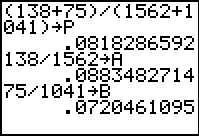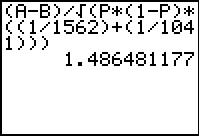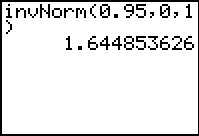••• ##### Device
• TI-83 Plus Family
• TI-84 Plus
• TI-84 Plus Silver Edition
•TI-84 Plus C Silver Edition
•TI-84 Plus CE

# Statistics: Claims About Two Proportions

by Texas Instruments#### Overview

Students test claims about two proportions by calculating test statistics, critical values, and P-values, for both one-tailed and two-tailed tests.

#### Key Steps

•In Problem 1, students discuss the test statistic that is used for testing a claim about two proportions. They test the claim that a greater proportion of women did not receive raises.

First they will write the null and alternative hypothesis for this right tailed test. Then students will find the two sample proportions and the total proportion.

•Students find their test statistic using the stored values of the proportions. They will compare this value to the critical value and use it to calculate the P-value later in the activity.

•Students are to find the critical value by using the invNorm command. They will use this value to determine if the test statistic is in the critical region and decide whether to reject or fail to reject the null hypothesis.